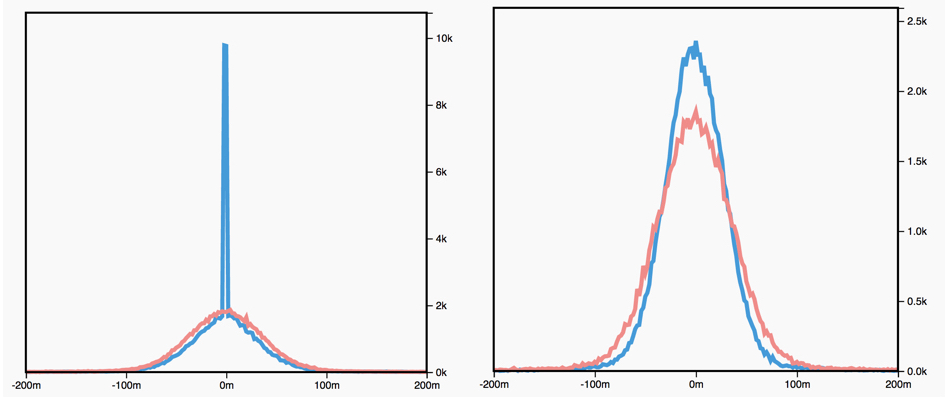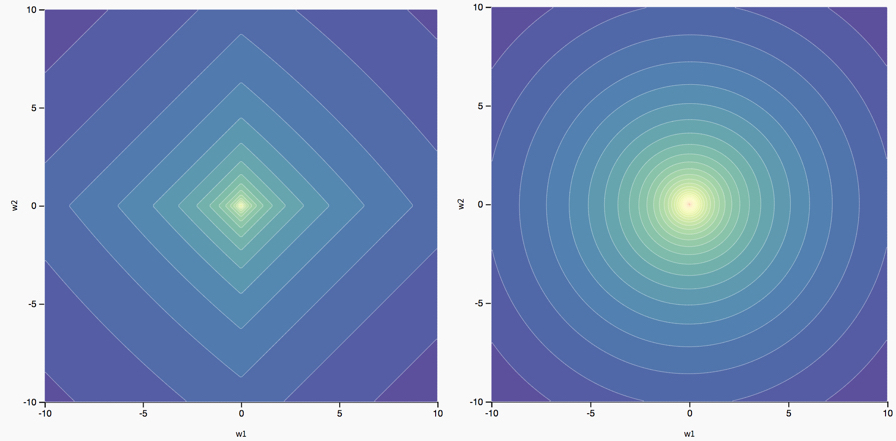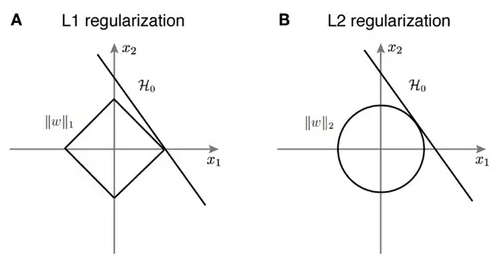## Introduction

• In order to avoid overfitting the training set (and thus improve generalization), you can try to reduce the complexity of the model by removing layers, and consequently decreasing the number of parameters. As shown in “A Simple Weight Decay Can Improve Generalization” by Krogh and Hertz (1992), another way to constrain a network and lower its complexity is to:

Limit the growth of the weights through some kind of weight decay.

• You want to prevent the weights from growing too large, unless it is really necessary. Intuitively, you are reducing the set of potential networks to choose from.

## Weight sparsity and why it matters

• Sparse vectors often contain many dimensions. Creating a feature-cross results in even more dimensions. Given such high-dimensional feature vectors, the model size may become huge and require huge amounts of RAM.

• In a high-dimensional sparse vector, it would be nice to encourage weights to drop to exactly 0 where possible. A weight of exactly 0 essentially removes the corresponding feature from the model. Zeroing out features will save RAM and may reduce noise in the model.

• For example, consider a housing dataset that covers the entire globe. Bucketing global latitude at the minute level (60 minutes per degree) gives about 10,000 dimensions in a sparse encoding; global longitude at the minute level gives about 20,000 dimensions. A feature-cross of these two features would result in roughly 200,000,000 dimensions. Many of those 200,000,000 dimensions represent areas of such limited residence (for example, the middle of the ocean) that it would be difficult to use that data to generalize effectively. It would be silly to pay the RAM cost of storing these unneeded dimensions. Therefore, it would be nice to encourage the weights for the meaningless dimensions to drop to exactly 0, which would allow us to avoid paying for the storage cost of these model coefficients at inference time.

• We might be able to encode this idea into the optimization problem done at training time, by adding an appropriately chosen regularization term.

• Would L2 regularization accomplish this task? Unfortunately not. L2 regularization encourages weights to be small, but doesn’t force them to exactly 0.0.

• An alternative idea would be to try and create a regularization term that penalizes the count of non-zero coefficient values in a model. Increasing this count would only be justified if there was a sufficient gain in the model’s ability to fit the data. Unfortunately, while this count-based approach is intuitively appealing, it would turn our convex optimization problem into a non-convex optimization problem. So this idea, known as L0 regularization, isn’t something we can use effectively in practice.

• However, there is a regularization term called L1 regularization that serves as an approximation to L0, but has the advantage of being convex and thus efficient to compute. So we can use L1 regularization to encourage many of the uninformative coefficients in our model to be exactly 0, and thus reap RAM savings at inference time.

## How does regularization work?

• L1 and L2 regularizations can be achieved by simply adding the corresponding norm term that penalizes large weights to the cost function. If you were training a network to minimize the cost $$J_{cross−entropy} = − \frac{1}{m} \sum\limits_{i = 1}^m {y^{(i)}}{log(\hat{y}^{(i)})}$$ with (let’s say) gradient descent, your weight update rule would be:
$w = w − \alpha \frac{\partial J_{cross−entropy}}{\partial w}$
• Instead, you would now train a network to minimize the cost

$J_{regularized} = J_{cross−entropy} + \lambda J_{L1\,or\,L2}$
• where,
• $$\lambda$$ is the regularization strength, which is a hyperparameter.
• $$J_{L1} = \sum\limits_{\text{all weights }w_k} | w_k |$$.
• $$J_{L2} = || w ||^2 = \sum\limits_{\text{all weights }w_k} | w_k |^2$$ (here, $$|| \cdot ||^2$$ is the L2 norm for vectors and the Frobenius norm for matrices).
• Your modified weight update rule would thus be:

$w = w − \alpha \frac{\partial J_{cross−entropy}}{\partial w} = w − \alpha (\frac{\partial J_{cross−entropy}}{\partial w} + \lambda \frac{\partial J_{L1\,or\,L2}}{\partial w})$
• For L1 regularization, this would lead to the update rule:
$w = w − \alpha \lambda sign(w) − \alpha \frac{\partial J_{cross−entropy}}{\partial w}$
• For L2 regularization, this would lead to the update rule:

$w = w − 2 \alpha \lambda w − \alpha \frac{\partial J_{cross−entropy}}{\partial w}$
• where,
• $$\alpha \lambda sign(w)$$ is the L1 penalty.
• $$2 \alpha \lambda w$$ is the L2 penalty.
• $$\alpha \frac{\partial J_{cross−entropy}}{\partial w}$$ is the gradient penalty.
• At every step of L1 and L2 regularization the weight is pushed to a slightly lower value because $$2 \alpha \lambda\,\ll\,1$$, causing weight decay.

• As seen above, the update rules for L1 and L2 regularization are different. While the L2 “weight decay” $$2w$$ penalty is proportional to the value of the weight to be updated, the L1 “weight decay” $$sign(w)$$ is not.

### Graphical treatment

• We know that $$L_1$$ regularization encourages sparse weights (many zero values), and that $$L_2$$ regularization encourages small weight values, but why does this happen?

• Let’s consider some cost function $$J(w_1,\dots,w_l)$$, a function of weight matrices $$w_1,\dots,w_l$$. Let’s define the following two regularized cost functions:

\begin{align} J_{L_1}(w_1,\dots,w_l) &= J(w_1,\dots,w_l) + \lambda\sum_{i=1}^l|w_i|\\ J_{L_2}(w_1,\dots,w_l) &= J(w_1,\dots,w_l) + \lambda\sum_{i=1}^l||w_i||^2 \end{align}
• Now, let’s derive the update rules for L1 and L2 regularization based on their respective cost functions.
• The update for $$w_i$$ when using $$J_{L_1}$$ is:
$w_i^{k+1} = w_i^{k} - \underbrace{\alpha\lambda sign(w_i)}_{L_1 \text{ penalty}} - \alpha\frac{\partial J}{\partial w_i}$
• The update for $$w_i$$ when using $$J_{L_2}$$ is:
$w_i^{k+1} = w_i^{k} - \underbrace{2\alpha\lambda w_i}_{L_2 \text{ penalty}}- \alpha\frac{\partial J}{\partial w_i}$
• The next question is: what do you notice that is different between these two update rules, and how does it affect optimization? What effect does the hyperparameter $$\lambda$$ have?
• The figure below shows a histogram of weight values for an unregularized (red) and L1 regularized (blue left) and L2 regularized (blue right) network:• The different effects of $$L_1$$ and $$L_2$$ regularization on the optimal parameters are an artifact of the different ways in which they change the original loss landscape. In the case of two parameters ($$w_1$$ and $$w_2$$), we can visualize this.

• The figure below shows the landscape of a two parameter loss function with L1 regularization (left) and L2 regularization (right):## L1 vs. L2 regularization

• L2 and L1 penalize weights differently:

• L2 penalizes $$weight^2$$.
• L1 penalizes $$|weight|$$.
• Consequently, L2 and L1 have different derivatives:

• The derivative of L2 is $$2 * weight$$.
• The derivative of L1 is $$k$$ (a constant, whose value is independent of the weight but depends on the sign of the weight).
• You can think of the derivative of L2 as a force that removes $$x\%$$ of the weight every time. As Zeno knew, even if you remove $$x\%$$ of a number billions of times, the diminished number will still never quite reach zero. (Zeno was less familiar with floating-point precision limitations, which could possibly produce exactly zero.) At any rate, L2 does not normally drive weights to zero.
• Simply put, for L2, the smaller the $$w$$, the smaller the penalty during the update of $$w$$ and vice-versa for larger $$w$$.

L2 regularization results in a uniform distribution of weights, as much as possible, over the entire weight matrix.

• You can think of the derivative of L1 as a force that subtracts some constant from the weight every time, irrespective of the weight’s magnitude. However, thanks to absolute values, L1 has a discontinuity at 0, which causes subtraction results that cross 0 to become zeroed out. For example, if subtraction would have forced a weight from +0.1 to -0.2, L1 will set the weight to exactly 0. Eureka, L1 zeroed out the weight!
• Simply put, for L1, note that while the penalty is independent of the value of $$w$$, but the direction of the penalty (positive or negative) depends on the sign of $$w$$.

L1 regularization thus results in an effect called “feature selection” or “weight sparsity” since it makes the irrelevant weights 0.

• L1 regularization – penalizing the absolute value of all the weights – turns out to be quite efficient for wide models.

• Note that this description is true for a one-dimensional model.

## Effect of L1 and L2 regularization on weights

• L1 and L2 regularizations have a dramatic effect on the weights values during training.

• For L1 regularization:
• “Too small” $$\lambda$$ constant: there’s no apparent effect.
• “Appropriate” $$\lambda$$ constant: many of the weights become zeros. This is called “sparsity of the weights”. Because the weight penalty is independent of the weight values, weights with value 0.001 are penalized just as much as weights with value 1000. The value of the penalty is $$\alpha \lambda$$ (generally very small). It constrains the subset of weights that are “less useful to the model” to be equal to 0. For example, you could effectively end up with 200 non-zero weights out of 1000, which means that 800 weights were less useful for learning the task.
• “Too large” $$\lambda$$ constant: You can observe a plateau, which means that the weights uniformly take values around zero. In fact, because $$\lambda$$ is large, the penalty −$$\alpha \lambda sign(w)$$ is much higher than the gradient −$$\alpha \frac{\partial J_{cross−entropy}}{\partial w}$$. Thus, at every update, $$w$$ is pushed by $$\approx −\alpha\lambda sign(w)$$ in the opposite direction of its sign. For instance, if w is around zero, but slightly positive, then it will be pushed towards −$$\alpha \lambda$$ when the penalty is applied. Hence, the plateau’s width should be $$2 \times \alpha \lambda$$.
• For L2 regularization:
• “Too small” $$\lambda$$ constant: there’s no apparent effect.
• “Appropriate” $$\lambda$$ constant: the weight values decrease following a centered distribution that becomes more and more peaked throughout training.
• “Too large” $$\lambda$$ constant: All the weights are rapidly collapsing to zeros, and the model obviously underfits because the weight values are too constrained.
• Note that the weight sparsity effect caused by L1 regularization makes your model more compact in theory, and leads to storage-efficient compact models that are commonly used in smart mobile devices.

## How does weight decay help the model generalize?

• Weight decay suppresses any irrelevant components of the weight vector by driving the optimization to find the smallest vector that solves the learning problem.
• Weight decay attenuates the influence of outliers on the weight optimization by constraining the weight values. There’s less risk for the weights to learn the sampling error (for example, when a subset of your data points comes from a wrong distribution or is mislabelled). In other words, the output of the model is less affected by changes in the input.
• L1 and L2 regularizations have a dramatic effect on the geometry of the cost function: adding regularization results in a more convex cost landscape and diminishes the chance of converging to a non-desired local minimum.

## Dropout regularization

• Although L1 and L2 regularization are simple techniques to reducing overfitting, there exist other methods, such as dropout regularization, that have been shown to be more effective at regularizing larger and more complex networks. If you had unlimited computational power, you could improve generalization by averaging the predictions of several different neural networks trained on the same task. The combination of these models will likely perform better than a single neural network trained on this task. However, with deep neural networks, training various architectures is expensive because:
• Tuning the hyperparameters is time consuming.
• Training the networks requires a lot of computations.
• You need a large amount of data to train the models on different subsets of the data.
• Dropout is a regularization technique, introduced in Srivastava et al. (2014), that allows you to combine many different architectures efficiently by randomly dropping some of the neurons of your network during training.

## Choosing L1-norm vs. L2-norm as the regularizing function

• In a typical setting, the L2-norm is better at minimizing the prediction error over the L1-norm. However, we do find the L1-norm being used despite the L2-norm outperforming it in almost every task, and this is primarily because the L1-norm is capable of producing a sparser solution.

• To understand why an L1-norm produces sparser solutions over an L2-norm during regularization, we just need to visualize the spaces for both the L1-norm and L2-norm.• In the diagram above, we can see that the solution for the L1-norm (to get to the line from inside our diamond-shaped space), it’s best to maximize $$x_1$$ and leave $$x_2$$ at 0, whereas the solution for the L2-norm (to get to the line from inside our circular-shaped space), is a combination of both $$x_1$$ and $$x_2$$. It is likely that the fit for L2 will be more precise. However, with the L1 norm, our solution will be more sparse.

• Since the L2-norm penalizes larger errors more strongly, it will yield a solution which has fewer large residual values along with fewer very small residuals as well.

• The L1-norm, on the other hand, will give a solution with more large residuals, however, it will also have a lot of zeros in the solution. Hence, we might want to use the L1-norm when we have constraints on feature extraction. We can easily avoid computing a lot of computationally expensive features at the cost of some of the accuracy, since the L1-norm will give us a solution which has the weights for a large set of features set to 0. A use-case of L1 would be real-time detection or tracking of an object/face/material using a set of diverse handcrafted features with a large margin classifier like an SVM in a sliding window fashion, where you would probably want feature computation to be as fast as possible in this case.

• Another way to interpret this is that L2-norm basically views all features similarly since it assumes all the features to be “equidistant” (given its geometric representation as a circle), while L1 norm views different features differently since it treats the “distance” between them differently (given its geometric representation as a square). Thus, if you are unsure of the kind of features available in your dataset and their relative importance, L2 regularization is the way to go. On the other hand, if you know that one feature matters much more than another, use L1 regularization.

• In summary,

• Broadly speaking, L1 is more useful for “what?” and L2 is more “how much?”.
• The L2 norm is as smooth as your floats are precise. It captures energy and Euclidean distance, things you want when, for e.g., tracking features. It’s also computation heavy compared to the L1 norm.
• The L1 norm isn’t smooth, which is less about ignoring fine detail and more about generating sparse feature vectors. Sparse is sometimes good e.g., in high dimensional classification problems.

## Conclusion

• When applying regularization methods, you need a metric to track your model’s improvement and generalization ability. The bias/variance tradeoff enables you to measure the efficiency of your regularization.
• Successfully training a model on complex tasks is complicated. You need to find a model architecture that can encompass the complexity of the dataset. Once you find such an architecture, you can work on improving generalization. Exploring, and even combining, different regularization techniques is an unmissable step of the training process. It helps you build intuition on the ability of a model to generalize in the real-world.

@article{Chadha2020DistilledRegularization,